## Plot multiple x-y relations with 3D-tilt

Dear community,

In a nutshell, I want to create a graph akin to this: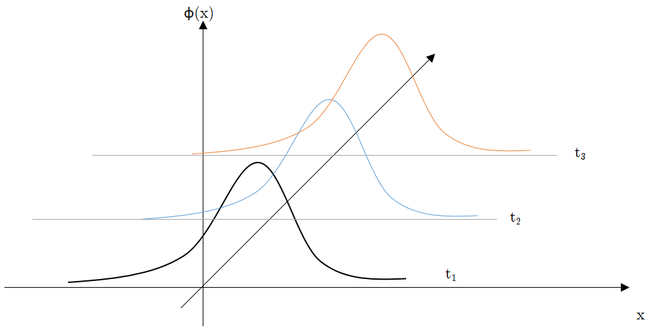.

That is, I have, as a data set, a discrete approximation of n probability-density functions (here, n=3).

I want to plot them such that they do not appear "on top of each other", but with a 3D-tilt.

Plotting "on top of each other" I achieved via proc gplot. However, I do not know how to tackle my current issue.

It would be great, if you could please provide help with respect to how achieve my goal.

Sample-Code is embedded in the spoiler;

Spoiler
data	in1;
input	x	t1 t2 t3;
datalines;
0.05	0.1	0.05	0.001
0.1	0.2	0.1	0.001
0.15	0.2	0.1	0.001
0.2	0.3	0.4	0.01
0.25	0.4	0.4	0.02
0.3	0.5	0.5	0.05
0.35	1	1	0.1
0.4	2	2	3
0.45	2.5	3	4
0.5	3	4.5	6
0.55	2.5	3	4
0.6	2	2	3
0.65	1	1	0.1
0.7	0.5	0.5	0.05
0.75	0.4	0.4	0.02
0.8	0.3	0.4	0.01
0.85	0.2	0.1	0.001
0.9	0.2	0.1	0.001
0.95	0.1	0.05	0.001
;
run;

symbol1
interpol		=			join
value			=			DiamondFilled
color			=			black
height			=			0.25;
symbol2
interpol		=			join
value			=			DiamondFilled
color			=			blue
height			=			0.25;
symbol3
interpol		=			join
value			=			DiamondFilled
color			=			orange
height			=			0.25;
axis1
label			=			("x")
minor			=			none
value			=			(color=black angle=45)
;
axis2
label			=			(angle=90 "\phi(x)")
minor			=			(n=1);
proc	gplot
data	=	in1
;
plot (t1 t2 t3)* x
/
overlay
haxis	=	axis1
vaxis	=	axis2
;
run; quit;



Yours sincerely,
Sinistrum

11 REPLIES 11

## Re: Plot multiple x-y relations with 3D-tilt

I might suggest Proc G3d. Use your current values as Z, add a Y value to create a different baseline for each distribution.

Then you have the Rotate and Tilt angle list options to create different appearance.

Instead of t1, t2, t3 etc, those would be Z axis dimension with Y=1 , 2, 3.

Something like this may get you started.

data plot;
set in1;
array t(*) t1-t3;
do y=1 to dim(t);
z= t[y];
output;
end;
keep x y z;
run;

proc g3d data=plot;
plot y*x=z /
rotate= (0 to 25 by 5)

;
run;
quit;

The Rotate is just an example that will generate 5 different graphs. You can play with different angles to get an appearance you like. Or Tilt. If you use both then pairs of angles from each list are used.

## Re: Plot multiple x-y relations with 3D-tilt

Thank you very much indeed.

Using

proc	g3d
data		=			plot
;
plot 		y * x = z
/
rotate	=	(315)
XYTYPE	=	2
CAXIS 	=	black
;
run;	quit;

, I am as far as follows, which is one step closer to the desired outcome: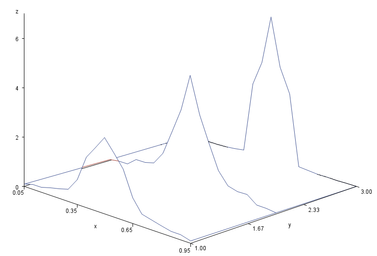.

Now, I need to consider, though cannot find ...

a way to change the colouring of the three different plots;

adjust point thickness (points so far are not displayed at all, just connecting lines).

It would be great, if, given someone has a clue on this, provide help once again, please.

## Re: Plot multiple x-y relations with 3D-tilt

First, let me ask the "tough love" questions ...

What question would you like for this graph to answer? ... And is a tilted 3d perspective graph the best way to answer those questions?

## Re: Plot multiple x-y relations with 3D-tilt

I did not want to clutter the post with too much of application-specific information / keep the code as "minimum-working-example-ish" as possible, though, given your question, I add more background; I am curious as to what you think and it certainly will help me to delineate my issues verbally.

This graph I would like to answer the question: "How do conditional density estimates develop over time, indexed by t + τ, for τ ranging from one to five, by one."

In my application, I obtain conditional density estimates, for a dependent variable, which lies τ periods ahead of the regressors in the regressor matrix.

Certain theories in "the" field imply certain stochastic processes for the dependent variable, suggesting a certain conditional distribution respectively a certain development towards something akin to an equilibrium state over time, given the regressors (admittedly, I am redundant with the "given the regressors", though, repetita iuvant).

In addition to (hopefully more rigorous) econometric tests, I would like to gauge by eye-balling, whether the estimates of densities I obtain give rise to the assumption that the specifications I implement are consistent with said theoretical considerations.

As τ ranges from one to five (as of now - it might well be for the interval's upper bound to increase in the wake of empirical analysis), plotting all densities on top of each other I consider as too cluttered (at least I am to dump to disentangle all the lines/colors/what not). Plotting them tilted, at least I hope and expect, would give a better feel on which regions of the density develop in which manner.DanH_sas
SAS Super FREQ

## Re: Plot multiple x-y relations with 3D-tilt

As an alternative to your original request, how about a comparative panel display like this: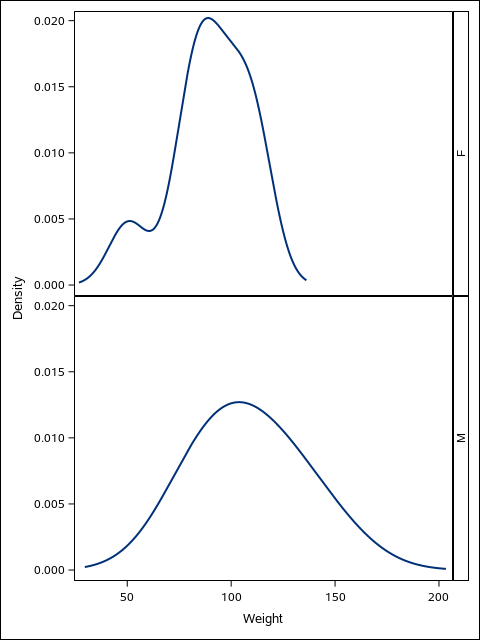You would stack all five density curves for easy comparison. The code for this would be something like the following:

ods graphics / reset width=480px height=640px imagename="Density";

proc sgpanel data=sashelp.class noautolegend;
panelby sex / layout=rowlattice onepanel novarname;
density weight / type=kernel;
/* series x=x y=y / smoothconnect; */
run;

In your case, you would use the SERIES statement instead of the DENSITY statement to display your results, as you have already pre-computed them. You would also substitute your "z" variable for the "sex" variable on the PANELBY statement. Use the WIDTH and HEIGHT options to control the aspect of the graph.

Hope this helps!

Dan

## Re: Plot multiple x-y relations with 3D-tilt

Thank you for this suggestion.

As an alternative for gplot, I am going to consider it.

Plotting strictly vertically, though, also imposes certain "spacing requirements".Ksharp
Super User

## Re: Plot multiple x-y relations with 3D-tilt

Calling @Rick_SAS

## Re: Plot multiple x-y relations with 3D-tilt

Depending on your application, you might want to look at the discussion and code at

https://blogs.sas.com/content/iml/2015/09/16/plot-distrib-exp.html

## Re: Plot multiple x-y relations with 3D-tilt

Thank you very much indeed (once again).

This quasi projects the z-axis to the plane; although I hope I would not have to end there, it brings me closer towards what I am looking for (in particular, I obtain the wanted tilt of the densities now).

Spoiler
data	in1;
input	x	t1 t2 t3;
datalines;
0.05	0.1	0.05	0.001
0.1	0.2	0.1	0.001
0.15	0.2	0.1	0.001
0.2	0.3	0.4	0.01
0.25	0.4	0.4	0.02
0.3	0.5	0.5	0.05
0.35	1	1	0.1
0.4	2	2	3
0.45	2.5	3	4
0.5	3	4.5	6
0.55	2.5	3	4
0.6	2	2	3
0.65	1	1	0.1
0.7	0.5	0.5	0.05
0.75	0.4	0.4	0.02
0.8	0.3	0.4	0.01
0.85	0.2	0.1	0.001
0.9	0.2	0.1	0.001
0.95	0.1	0.05	0.001
;
run;
%macro	rearranger1;
%DO		i	=	1	%TO			3;
data	_&i.
;
set		in1
(
keep		=
x
t&i.
rename		=
(
t&i.		=		y
)

)
;
s	=	&i.	;
run;
%END;
data	in2
;
set
_:
;
run;
proc	sql		noprint;
select
max(y)	as	maxy
into
:maxy
separated	by	' '
from
in2
;
quit;
data	in3
;
set
in2
;
s	=	s	*	ceil(&maxy.);
y	=	y	+	s;
run;

%mend	rearranger1;
%rearranger1;
proc	sgplot
data	=		in3
noautolegend
;
band
y			=			x
lower		=			s
upper		=			y
/
group		=			s
;
run;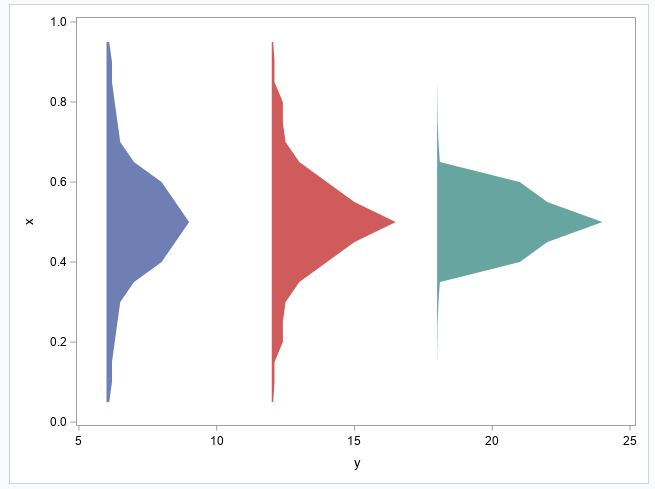## Re: Plot multiple x-y relations with 3D-tilt

Thank you so much - this is exciting.

If I managed to get this running, I assume, I can give up the "tilt" and plot the densities that way.

I am missing just one step, as I am too dumb to figure out how to plot the densities exactly on the corresponding lines (they are shifted upwards).

Spoiler
data	in1;
input	x	t1 t2 t3;
datalines;
0.05	0.1	0.05	0.001
0.1	0.2	0.1	0.001
0.15	0.2	0.1	0.001
0.2	0.3	0.4	0.01
0.25	0.4	0.4	0.02
0.3	0.5	0.5	0.05
0.35	1	1	0.1
0.4	2	2	3
0.45	2.5	3	4
0.5	3	4.5	6
0.55	2.5	3	4
0.6	2	2	3
0.65	1	1	0.1
0.7	0.5	0.5	0.05
0.75	0.4	0.4	0.02
0.8	0.3	0.4	0.01
0.85	0.2	0.1	0.001
0.9	0.2	0.1	0.001
0.95	0.1	0.05	0.001
;
run;
%macro	rearranger1;
%DO		i	=	1	%TO			3;
data	_&i.
;
set		in1
(
keep		=
x
t&i.
rename		=
(
t&i.		=		y
)

)
;
s	=	&i.	;
run;
%END;
data	in2
;
set
_:
;
run;
proc	sql		noprint;
select
max(y)	as	maxy
into
:maxy
separated	by	' '
from
in2
;
quit;
data	in3
;
set
in2
;
s0	=	s;
s	=	s	*	ceil(&maxy.);
y	=	y	+	s;
run;

%mend	rearranger1;
%rearranger1;

%LET		scaling	=		0.1;
data	in4;
set			in3;
y_with_offset	=	s0	-	(y*&scaling);
run;

proc sgplot
data				=			in4
;
band
x				=			x
upper			=			3
lower			=			y_with_offset
/
group			=			s0
;
yaxis
display			=
(
noline
noticks
nolabel
)
reverse
offsetmin		=		.1
values			=
(
0 to 3 by 1
)
valuesdisplay	=
(
'' '1' '2' '3'
)
;
xaxis
display			=
(
noline
)
values			=
(
0 to 1.0 by .1
)
;
run; quit;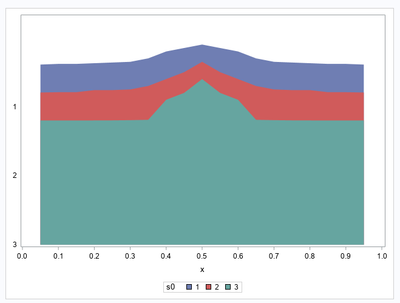Discussion stats
• 11 replies
• 655 views
• 8 likes
• 6 in conversation